# Cyclic Permutations

Consider the permutations of 3 elements: 123, 132, 213, 231, 312, 321. One way to visualize the 3! different permutations is to draw a dot for each element, and then draw an arrow joining adjacent elements: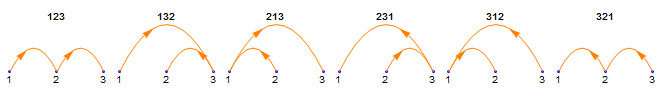Each of the diagrams can be turned into a loop by drawing another arrow that joins the last element in a permutation with the first one: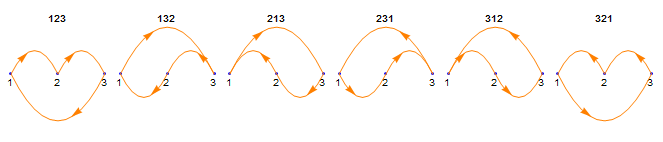Next, take the dots and arrange them evenly around a circle: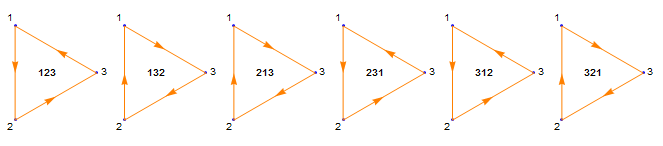If only the shape of a diagram matters—since a loop doesn’t have a particular beginning or end—each figure is either a counterclockwise arrow (123, 231, and 312) or a clockwise arrow (132, 213, and 321) around the points: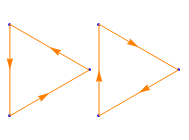So far, these correspond to the Stirling numbers of the first kind s(n, 1).

And if the direction of the arrows doesn’t matter, there’s only one shape: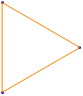This is what you’d get if you stretched a rubber band around n nails spaced evenly around a circle.

For n points, the number of distinct loops is n!/(2n). To begin, the number of permutations of n elements is n!. When the first and last elements are joined to make a loop, the overall count is divided by n because each loop is represented by n different permutations, one starting at each of the points: 123, 231, and 312 are equivalent counterclockwise loops, and 132, 213, and 321 are equivalent clockwise loops.

Moreover, because each loop has a clockwise representation and a counterclockwise representation, we can further divide the count by 2 when orientation no longer matters.

For greater values of n, then, more shapes are possible. For example, there are 3 basic ways to twist a rubber band around 4 nails, corresponding to 4!/(2·4):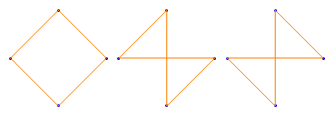For 5 nails, 12 shapes: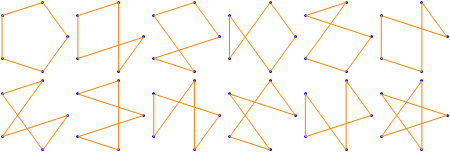And for 6 nails, 60 shapes: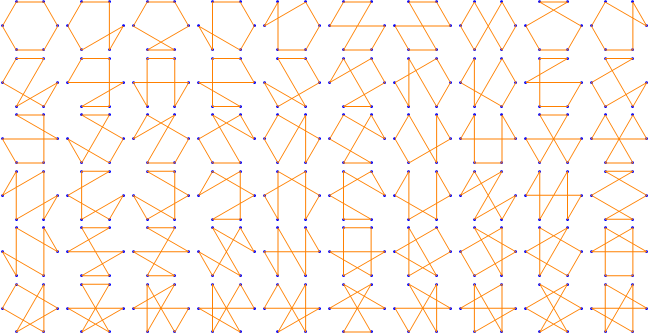The same reasoning applies to counting necklaces or bracelets made up of n distinct beads, where the points change places for each permutation but the overall shape is always a circle.

This is sequence A001710 at the Online Encyclopedia of Integer Sequences.

See G. E. Andrews, Number Theory, Dover Publications: New York, 1994, pp. 38–40, where the shapes are called stellated p-gons.

Designed and rendered using Mathematica 9.